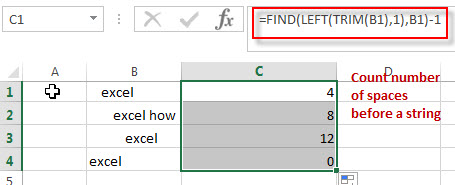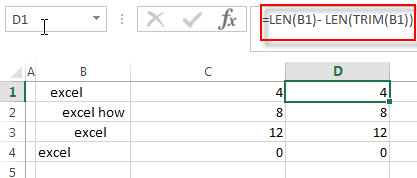# How to Count Spaces before the Text String

This post will guide you how to count the number of spaces before the text string in a cell in Excel. How to count the leading spaces before the text string in one cell with formula in excel. How do I create an excel formula that counts the number of spaces before the text string.

## Count number of spaces before a string

Assuming that you have lots of cells contain text string with different levels of indentation on them. You want to count the number of spaces before each test string in cells, and then you want to know how to count the number of spaces before the text string in each cell.

You can create an excel formula based on the FIND function, the LEFT function, and the TRIM function.

For example, let’s count the number of spaces before text string in Cell B1, you can write down the following excel formula:

`=FIND(LEFT(TRIM(B1),1)B1)-1`Let’s see how this formula works:

The TRIM function can be used to remove all spaces at the start or end of the text string, then you can get a text string without any spaces. The returned result will pass into the LEFT function to get the leftmost characters of the result without spaces.

Then use the FIND function to search for that characters in the original string, and it returns the position of the first character of the searching characters in the original string. The result is subtracted 1 to get the number of spaces before the text string in Cell B1.

When you use this formula, you do not need to care how many other spaces there are or where they are.

If there are no spaces at the end of the text string in each cell, then you also can use the following formula:

`=LEN(B1)- LEN(TRIM(B1))`### Related Formulas

• Get first word from text string
If you want to extract the first word from a text string in a cell, you can use a combination of the IF function, the ISERR function, the LEFT function and the FIND function to create a complex excel formula..…
• Get last word from text string
If you want to get the last word from a text string, you can create an excel formula based on the RIGHT function, the LEN function, the FIND function and the SUBSTITUTE function..…
• Extract nth word from text string
If you want to extract the nth word from a text string in a single cell, you can create an excel formula based on the TRIM function, the MID function, the SUBSTITUTE function, the REPT function and the LEN function..…
• count specific words in a cell or a range
If you want to count the number of a specific word in a single cell, you need to use the SUBSTITUTE function to remove all that certain word in text string, then using LEN function to calculate the length of the substring that without that specific word.…

### Related Functions

• Excel LEFT function
The Excel LEFT function returns a substring (a specified number of the characters) from a text string, starting from the leftmost character.The LEFT function is a build-in function in Microsoft Excel and it is categorized as a Text Function.The syntax of the LEFT function is as below:= LEFT(text,[num_chars])…t)…
• Excel FIND function
The Excel FIND function returns the position of the first text string (sub string) within another text string.The syntax of the FIND function is as below:= FIND(find_text, within_text,[start_num])…
• Excel LEN function
The Excel LEN function returns the length of a text string (the number of characters in a text string).The syntax of the LEN function is as below:= LEN(text)…
• Excel TRIM function
The Excel TRIM function removes all spaces from text string except for single spaces between words.  You can use the TRIM function to remove extra spaces between words in a string.The syntax of the TRIM function is as below:= TRIM (text)….

Related Posts
How to Remove All Extra Spaces and Keep Only One Between Words in Excel

Sometimes when copying something from other type files to excel, there might be two or more spaces display between words. Extra spaces between words are frequently to be seen in excel, and this behavior is very annoying. If we want ...

How to Remove Middle Name from Full Name in Excel

When we get a list of full names, we can remove the middle name for short in some cases. As the middle names are different among the full name list so we cannot replace them by space directly in excel. ...

How to Count Comma Separated Value in One Cell in Excel

This post will guide you how to count values in a single cell separated by commas with a formula in Excel. How do I count comma separated values in one cell in Excel 2013/2016. Is it possible to have a ...

How to Remove the First/Last Word from Text string in Cell

This post will guide you how to remove the first and the last word from a text string in cells in Excel. How do I use a formula to remove first and last word of a text string in Excel. ...

This post will guide you how to extract email address from a text string in Excel. How do I use a formula to extract email address in Excel. How to extract email address from text string with VBA Macro in ...

Remove Trailing Spaces from Cells in Excel

This post will guide you how to remove leading and trailing spaces in cells in Excel. How do I remove leading or trailing spaces in a range of cells with a formula in Excel. How to remove trailing spaces from ...

Convert Scientific Notation to Text or a Number in Excel

This post will guide you how to convert scientific notation to text or a number in Excel. How do I convert excel scientific to text with a formula in Excel. Convert Scientific Notation to Text or a Number in Excel ...

Reverse Concatenate Formula in Excel

This post will guide youw how to reverse the concatenate function in Excel. how do I split the text string into the muliptle cells with a formula in Excel. How to split text data of a cell to multiple cells ...

Remove Spaces from a String

This post will guide you how to remove extra spaces between words or text string from the specified range of cells in excel. How to delete all spaces from excel cells. For example, when you paste data from an external ...